# VAE生成模型（附VAE实现mnist代码）

【学习笔记】生成模型——变分自编码器

# 自编码器AE

AutoEncoder 包括 编码器(Encoder) 和 解码器(Decoder) 两部分。Encoder 过程是将原先的数据（常用于图像方向）压缩为低维向量；Decoder 则是把低维向量还原为原来数据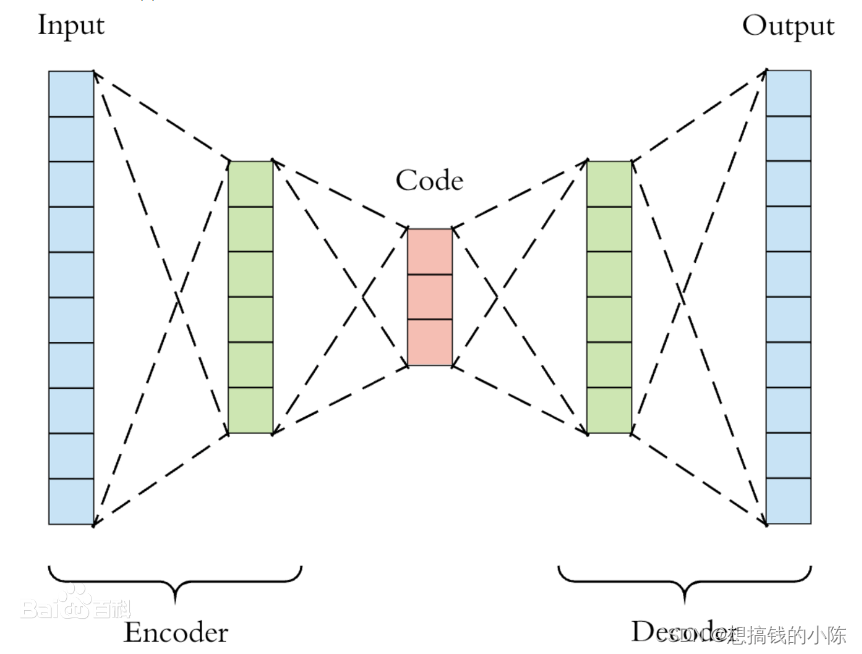# 变分自编码（Variational AutoEncoder）

## 定义

VAE 模型是一种包含隐变量的生成模型，它利用神经网络训练得到两个函数（也称为推断网络和生成网络），进而生成输入数据中不包含的数据。## 隐变量

• 隐变量 z 是可以认为是隐藏层数据，它是不限定数目的符合 高斯分布 特征的数据。（根据实际情况确定数目）
• z zz 由输入数据 X XX 的采样以及参数生成，它既包含 X XX 的信息（这个于 AutoEncoder 的隐藏层类似），同时也满足 高斯分布，方便接下来进行梯度下降或者其他优化技术（By having a Gaussian distribution, we can use gradient descent (or any other optimization technique) to increase P ( X ) P(X)P(X) by making f ( z ; θ ) f(z; \theta)f(z;θ) approach X XX for some z zz ,i.e., gradually making the training data more likely under the generative model.）。
• 隐变量的作用除了让生成网络尽可能还原原来的数据 X，同时也能生成原来数据中不存在的数据。
• ## VAE的模型架构上面这张图就是VAE的模型架构，我们先粗略地领会一下这个模型的设计思想。

在auto-encoder中，编码器是直接产生一个编码的，但是在VAE中，为了给编码添加合适的噪音，编码器会输出两个编码，一个是原有编码(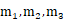)，另外一个是控制噪音干扰程度的编码()，第二个编码其实很好理解，就是为随机噪音码(e1,e2,e3)分配权重，然后加上exp(σi)的目的是为了保证这个分配的权重是个正值，最后将原编码与噪音编码相加，就得到了VAE在code层的输出结果(c1,c2,c3)。其它网络架构都与Deep Auto-encoder无异。

损失函数方面，除了必要的重构损失外，VAE还增添了一个损失函数（见上图Minimize2内容），这同样是必要的部分，因为如果不加的话，整个模型就会出现问题：为了保证生成图片的质量越高，编码器肯定希望噪音对自身生成图片的干扰越小，于是分配给噪音的权重越小，这样只需要将(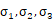)赋为接近负无穷大的值就好了。所以，第二个损失函数就有限制编码器走这样极端路径的作用，这也从直观上就能看出来，exp(σi)-(1+σi)在σi=0处取得最小值，于是(σi)就会避免被赋值为负无穷大。

上述我们只是粗略地理解了VAE的构造机理，但是还有一些更深的原理需要挖掘，例如第二个损失函数为何选用这样的表达式，以及VAE是否真的能实现我们的预期设想，即“图片能够编码成易于表示的形态，并且这一形态能够尽可能无损地解码回原真实图像”，是否有相应的理论依据。

下面我们会从理论上深入地分析一下VAE的构造依据以及作用原理。

## VAE原理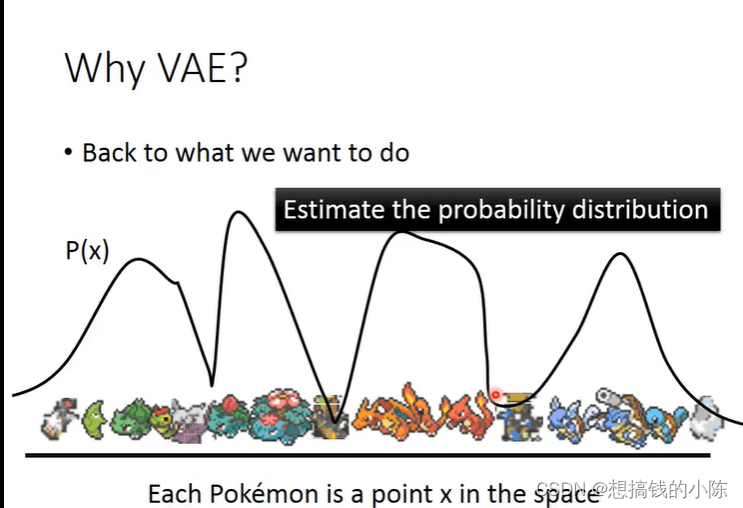这里以李宏毅的视频为例。假设我们的输入图像的特征是X，我们希望通过P（X）采样得到一个分布，这个分布反映了真实数据的分布。我们就是需要估计出这个分布。P（X）可以看作是一个高斯混合分布（可以理解为任意的数据分布，都可以由若干的m个高斯分布组成），其公式如下：

P（m）是取到第m个高斯分布的概率，P（X|m）是第m个高斯分布，如下图所示。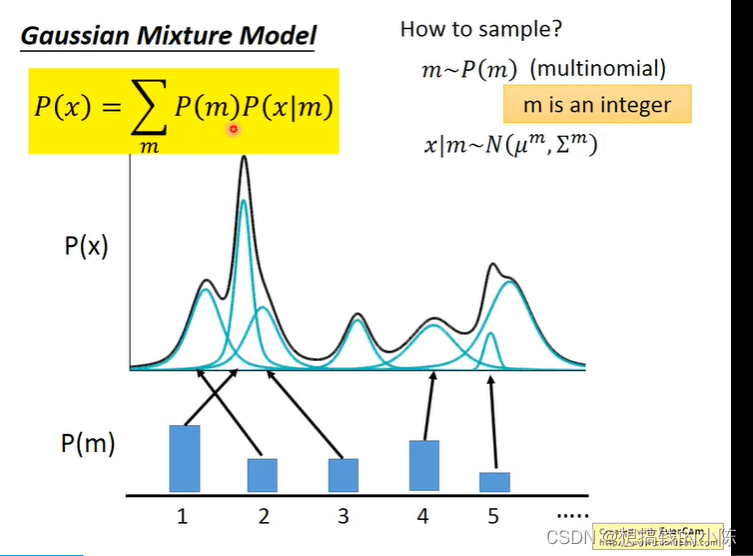但现实情况下，P（X）通常是由无限个的高斯组成的，以上离散的方式只是存在于限制，因此，将其变为积分的形式，代表由infite的高斯分布组成。公式如：（这里我把m用字母z表示）。其中，

• P（z）是已知的，z~N(0,1)（实际上并不一定要选用（0,1），其他的连续分布都是可行的）可以理解为就是encoder中decoder中的中间变量，但vae将其称之为数据的分布，因为encoder出的不是特征，而是数据的均值和方差。
• P（x|z）是未知的。x|z~N(u(z), σ(z))。
• 于是，我们真正需要求解的，是u(z)和σ（z）2个函数的表达式。但又因为P（x）通常非常复杂，导致u（z）和sigma(z）都难以计算，所以引入2个神经网络(encoder和decoder)来帮助我们求解。

第一个神经网络叫做Decoder，它求解的是u和σ两个函数，这等价于求解P(x|z)。第二个神经网络叫做encoder，它求解的是结果是q(z|x)，q可以代表任何分布。值得注意的是，这儿引入第二个神经网路Encoder的目的是，辅助第一个Decoder求解p(x|z)，这也是整个VAE理论中最精妙的部分，下面我会详细地解释其中的奥妙。

我们先回到最开始要求解的目标式：

我们希望P(x)越大越好，这等价求解（我理解为是最大化似然函数）：

注意到

q(z|x)可以是任何分布，这个式子从左边到右边就是多了一项积分，这个积分的和为1。

对公式1继续展开，

上面的第二项是一个大于等于0的值，于是我们就找到了一个logP（x）的下界：

我们把这个下界记为Lb，上面的式子就可以化为

原本我们需要求P(x|z)使得logP(x)最大，现在引入了一个q(z|x)，变成了同时求P（x|z）和q(z|x）使得logP(x)最大，不妨观察一下logP(x)和Lb的关系：一个有趣的现象是，当我们固定住P（x|z）时，因为logP(x)只与P(x|z)有关，所以logP(x)的值是会不变的，此时我们去调节q(z|x)，使得Lb越来越高，同时KL散度越来越小，当我们调节到q(z|x)与P(z|x)完全一致时，KL散度就消失为0，Lb与logP(x)完全一致。由此可以得出，不论logP(x)的值如何，我们总能够通过调节使得Lb等于logP(x)，又因为Lb是logP(x)的下界，所以求解Maximum logP(x)等价为求解Maximum Lb。

这个现象从宏观上来看也是很有意思，调节P(x|z)就是在调节decoder，调节q(z|x)就是在调节encoder。于是，VAE的训练逻辑就变成了decoder每前进一步，encoder就调节成与其一致的样子，并且站在那拿“枪”顶住decoder，这样在下次训练的时候decoder就只能前进，不能退步了。

上述便是VAE的巧妙设计之处。再回到我们之前的步骤上，现在需求解Maximum Lb。

注意到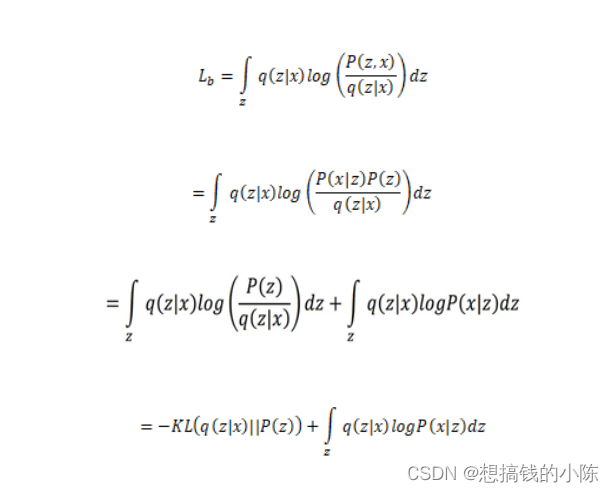所以，求解Maximum Lb，等价于求解KL的最小值和q(z|x)logP（x|z）dz的最大值。

我们先来求第一项，其实KL的展开式刚好等于：具体的展开计算过程可以参阅《Auto-Encoding Variational Bayes》的Appendix B。

于是，第一项式子就是第二节VAE模型架构中第二个损失函数的由来。

接下来求第二项，注意到上述的这个期望，也就是表明在给定q(z|x)（编码器输出）的情况下P（x|z）（解码器输出）的值尽可能高，这其实就是一个类似于Auto-Encoder的损失函数（方差忽略不计的话）：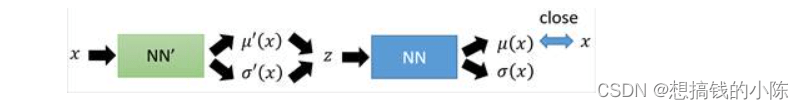因此，第二项式子就是第二节VAE模型架构中第一个损失函数的由来。

综上，关于VAE模型架构中的理论证明部分至此全部介绍完毕。

# 代码

## 导入相关包

#%% 导入包
from AutoEncoder import *
import torch
from torchvision import transforms
from torchvision.datasets import MNIST
from torchvision.utils import save_image
import matplotlib.pyplot as plt

## 下载数据集，并查看前6张图片

#%%
img_transform = transforms.Compose([
transforms.ToTensor()])
path = "../../dataset"
batch_size = 128
imgs = dataset.data[:6].numpy()
labels = dataset.targets[:6].numpy()

_, axes = plt.subplots(2, 3)
for i in range(2):
for j in range(3):
axes[i][j].imshow(imgs[i*3 + j], cmap='gray')
axes[i][j].set_title("True: " + str(labels[i*3+j]))
axes[i][j].get_xaxis().set_visible(False)
axes[i][j].get_yaxis().set_visible(False)
plt.show()

## 定义VAE模型

class VAE(nn.Module):
# 使用全链接网络
def __init__(self, encoder_structure, decoder_structure, hidden_num):
super(VAE, self).__init__()
self.encoder = nn.Sequential()
for i in range(1, len(encoder_structure)):

self.z_layer = nn.Linear(encoder_structure[-1], hidden_num)
self.log_var_layer = nn.Linear(encoder_structure[-1], hidden_num)

self.decoder = nn.Sequential()
for i in range(1, len(decoder_structure)):

def forward(self, x):
self.z_mean, self.z_log_var = self.encode(x)
z = self._reparameters(self.z_mean, self.z_log_var)
self.x_mean = self.decode(z)
return self.z_mean, self.z_log_var, z, self.x_mean

def encode(self, x):
code = self.encoder(x)
z_mean = self.z_layer(code)
z_log_var = self.log_var_layer(code)
return z_mean, z_log_var

def decode(self, z):
x_mean = self.decoder(z)
return x_mean

def loss(self, x, recon_func):
KL_loss = -0.5 * torch.sum(1 + self.z_log_var - self.z_mean.pow(2) - self.z_log_var.exp())
recon_loss = recon_func(self.x_mean, x)
return KL_loss + recon_loss

def _reparameters(self, z_mean, z_log_var):
z0 = torch.randn_like(z_mean)
return z_mean + z0 * torch.exp(0.5*z_log_var)

def train(self, net, dataIter, recon_loss, optimizer, epoches):
device = torch.device('cuda' if torch.cuda.is_available() else 'cpu')
print("training on %s" %(device))
net = net.to(device)
train_loss = [0.]*epoches
for epoch in range(epoches):
cnt = 0
for batch_idx, (data, label) in enumerate(dataIter):
# 前向
data = data.view(data.size(0), -1).to(device)
z_mean, z_log_var, z, x_mean = net(data)
loss = net.loss(data, recon_loss)
# 反向
loss.backward()
optimizer.step()
train_loss[epoch] += loss.cpu().item()
if((batch_idx+1) % 100 == 0):
print("epoch : {0} | #batch : {1} | batch average loss: {2}"
.format(epoch, batch_idx, loss.cpu().item()/len(data)))
# train_loss[epoch] /= len(dataIter.dataset)
print("Epoch : {0} | epoch average loss : {1}"
.format(epoch, train_loss[epoch] / len(dataIter.dataset)))

## 定义模型并训练

encoder_structure = [784, 512, 64]
decoder_structure = [20, 64, 512, 784]
model = VAE(encoder_structure, decoder_structure, 20)
print(model)
model.train(model, dataIter, nn.MSELoss(size_average=False), opt, 50)

## 随机采样几个编码，并生成样本

shape = (6, 20)
z_mean = torch.rand(shape, device='cuda')

rand_z = torch.randn(shape,device='cuda') + z_mean
gen_x = model.decode(rand_z).cpu()
rand_img = to_image(gen_x).detach().numpy()
# rand_img = (rand_img * 255 / (rand_img.max() - rand_img.min())).astype(np.uint8)
_ ,axes = plt.subplots(2, 3)
for i in range(2):
for j in range(3):
axes[i][j].imshow(rand_img[i*3+j], cmap='gray')
plt.show()